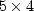Next: Preconditioning Up: ITERATIVE METHODS Previous: Conjugate-gradient theory for programmers

The conjugate-gradient program can be divided into two parts: an inner part that is used almost without change over a wide variety of applications, and an outer part containing the initializations. Since Fortran does not recognize the difference between upper- and lower-case letters, the conjugate vectors G and S in the program are denoted by gg and ss. The inner part of the conjugate-gradient task is in subroutine cgstep().

# A step of conjugate-gradient descent.
#
subroutine cgstep( iter,   n, x, g, s,   m, rr, gg, ss)
integer i,         iter,   n,            m
real    x(n), rr(m)     # solution, residual
real    s(n), ss(m)     # step,     conjugate step
real dot, sds, gdg, gds, determ, gdr, sdr, alfa, beta
if( iter == 0 ) {
do i= 1, n
s(i) = 0.
do i= 1, m
ss(i) = 0.
if( dot(m,gg,gg)==0 )   call erexit('cgstep: grad vanishes identically')
alfa =  dot(m,gg,rr) / dot(m,gg,gg)
beta = 0.
}
else {                          # search plane by solving 2-by-2
gdg = dot(m,gg,gg)      #  G . (R - G*alfa - S*beta) = 0
sds = dot(m,ss,ss)      #  S . (R - G*alfa - S*beta) = 0
gds = dot(m,gg,ss)
determ = gdg * sds - gds * gds + (.00001 * (gdg * sds) + 1.e-15)
gdr = dot(m,gg,rr)
sdr = dot(m,ss,rr)
alfa = ( sds * gdr - gds * sdr ) / determ
beta = (-gds * gdr + gdg * sdr ) / determ
}
do i= 1, n                                      # s = model step
s(i)  = alfa * g(i)  + beta * s(i)
do i= 1, m                                      # ss = conjugate
ss(i) = alfa * gg(i) + beta * ss(i)
do i= 1, n                                      # update solution
x(i)  =  x(i) +  s(i)
do i= 1, m                                      # update residual
rr(i) = rr(i) - ss(i)
return; end

real function dot( n, x, y )
integer i, n;           real val, x(n), y(n)
val = 0.;       do i=1,n  { val = val + x(i) * y(i) }
dot = val;      return;         end


This program was used to produce about 50 figures in this book. The first example of its use is the solution of theset of simultaneous equations below. Observe that the exact'' solution is obtained in the last step. Since the data and answers are integers, it is quick to check the result manually.

y transpose
3.00      3.00      5.00      7.00      9.00

A transpose
1.00      1.00      1.00      1.00      1.00
1.00      2.00      3.00      4.00      5.00
1.00      0.00      1.00      0.00      1.00
0.00      0.00      0.00      1.00      1.00
for iter = 0, 4
x    0.43457383  1.56124675  0.27362058  0.25752524
res  0.73055887 -0.55706739 -0.39193439  0.06291389  0.22804642
x    0.51313990  1.38677311  0.87905097  0.56870568
res  0.22103608 -0.28668615 -0.55250990  0.37106201  0.10523783
x    0.39144850  1.24044561  1.08974123  1.46199620
res  0.27836478  0.12766024 -0.20252618  0.18477297 -0.14541389
x    1.00001717  1.00006616  1.00001156  2.00000978
res -0.00009474 -0.00014952 -0.00022683 -0.00029133 -0.00036907
x    0.99999994  1.00000000  1.00000036  2.00000000
res -0.00000013 -0.00000003  0.00000007  0.00000018 -0.00000015


Initialization of the conjugate-gradient method typically varies from one application to the next, as does the setting up of the transformation and its adjoint. The problem above was set up with the matmul() program given in chapter. The program cgmeth() below initializes a zero solution and the residual of a zero solution.

# setup of conjugate gradient descent, minimize  SUM rr(i)**2
#                   nx
# rr(i)  = yy(i) - sum aaa(i,j) * x(j)
#                  j=1
subroutine cgmeth( nx,x, nr,yy,rr, aaa, niter)
integer i, iter,   nx,   nr,            niter
real    x(nx), yy(nr), rr(nr), aaa(nr,nx)
temporary real dx(nx), sx(nx), dr(nr), sr(nr)
do i= 1, nx
x(i) = 0.
do i= 1, nr
rr(i) = yy(i)
do iter= 0, niter {
call matmult( 1, aaa, nx,dx, nr,rr)              # dx= dx(aaa,rr)
call matmult( 0, aaa, nx,dx, nr,dr)              # dr= dr(aaa,dx)
call cgstep( iter, nx, x,dx,sx, _
nr,rr,dr,sr)                 # x=x+s; rr=rr-ss
}
return; end


Then it loops over iterations, invoking matrix multiply, conjugate transpose multiply, and the conjugate-gradient stepper. In subroutine cgmeth(), the variable dx is like g in equation (44), and the variable dr is like G in equation (45).Next: Preconditioning Up: ITERATIVE METHODS Previous: Conjugate-gradient theory for programmers
Stanford Exploration Project
10/21/1998